Study Materials: ncert solutions

Our ncert solutions for Exercise 2.4 Class 9 maths 2. Polynomials - ncert solutions - Toppers Study is the best material for English Medium students cbse board and other state boards students.

Solutions ⇒ Class 9th ⇒ Mathematics ⇒ 2. Polynomials

# Exercise 2.4 Class 9 maths 2. Polynomials - ncert solutions - Toppers Study

Topper Study classes prepares ncert solutions on practical base problems and comes out with the best result that helps the students and teachers as well as tutors and so many ecademic coaching classes that they need in practical life. Our ncert solutions for Exercise 2.4 Class 9 maths 2. Polynomials - ncert solutions - Toppers Study is the best material for English Medium students cbse board and other state boards students.

## Exercise 2.4 Class 9 maths 2. Polynomials - ncert solutions - Toppers Study

CBSE board students who preparing for class 9 ncert solutions maths and Mathematics solved exercise chapter 2. Polynomials available and this helps in upcoming exams 2023-2024.

### You can Find Mathematics solution Class 9 Chapter 2. Polynomials

• All Chapter review quick revision notes for chapter 2. Polynomials Class 9
• NCERT Solutions And Textual questions Answers Class 9 Mathematics
• Extra NCERT Book questions Answers Class 9 Mathematics
• Importatnt key points with additional Assignment and questions bank solved.

NCERT Solutions do not only help you to cover your syllabus but also will give to textual support in exams 2023-2024 to complete exercise 2.4 maths class 9 chapter 2 in english medium. So revise and practice these all cbse study materials like class 9 maths chapter 2.4 in english ncert book. Also ensure to repractice all syllabus within time or before board exams for ncert class 9 maths ex 2.4 in english.

See all solutions for class 9 maths chapter 2 exercise 2 in english medium solved questions with answers.

### Exercise 2.4 class 9 Mathematics Chapter 2. Polynomials

• Exercise 2.4 Class 9 Maths 2. Polynomials - Ncert Solutions - Toppers Study
• Class 9 Ncert Solutions
• Solution Chapter 2. Polynomials Class 9
• Solutions Class 9
• Chapter 2. Polynomials Exercise 2.4 Class 9

## Exercise 2.4 Class 9 maths 2. Polynomials - ncert solutions - Toppers Study

Chapter 2. Polynomials

Exercise 2.4

Q.1. Determine which of the following polynomials has (x+ 1) a factor:

(i) x+x+ x +1                                        (ii) x+ x+ x+ x +1

(iii) x+ 3x+ 3x+ x +1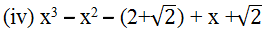Solution:

(i) If (x + 1) is a factor of p(x) = x3+ x2+ x + 1, then p (−1) must be zero, otherwise (x + 1) is not a factor of p(x).

P(x)  = x+ x+ x + 1

P(−1)= (−1)+ (−1)+ (−1) + 1

= − 1 + 1 − 1 − 1

= 0

∵ P(x) = 0

Hence, x + 1 is a factor of this polynomial.

(ii) If (x + 1) is a factor of p(x) = x4+ x3+ x2+ x + 1, then p (−1) must be zero, Otherwise (x + 1) is not a factor of p(x).

P(x) = x4+ x3+ x2+ x + 1

P(−1) = (−1)+ (−1)+ (−1)+ (−1) + 1

= 1 − 1 + 1 −1 + 1

= 1

As P(x) ≠ 0, (− 1)

Therefore, x + 1 is not a factor of this polynomial.

(iii) If (x + 1) is a factor of polynomial p(x) = x4+ 3x3+ 3x2+ x + 1, then p (−1) must  be 0, otherwise (x + 1) is not a factor of this polynomial.

P(x) = x4+ 3x3+ 3x2+ x + 1

P(−1) = (−1)4+ 3(−1)3+ 3(−1)2+ (−1) + 1

= 1 − 3 + 3 − 1 + 1

= 1

As P(x) ≠ 0, (−1)

Therefore (x+1) is not a factor of this polynomial .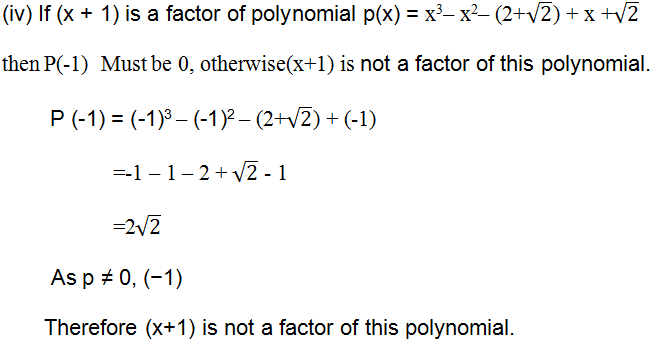Q.2. Use the Factor Theorem to determine whether g(x) is a factor of p(x) in each of the following cases:

(i)         P(x) = 2x3+ x2− 2x − 1, g(x) = x + 1

(ii)        P(x) = x+ 3x+ 3x + 1, g(x) = x + 2

(iii)       P(x) = x− 4 x+  x + 6, g(x) = x − 3

Solution:

(i)   If g(x) = x + 1 is a factor of the given polynomial p(x), then p (−1) must be zero.

P (x) = 2x+ x− 2x − 1

P (−1) = 2(−1)3+ (−1)2− 2(−1) − 1

= 2(−1) + 1 + 2 – 1

= 0

∵ P(x) = 0

Hence, g(x) = x + 1 is a factor of the given polynomial.

(ii) If g(x) = x + 2 is a factor of the given polynomial p(x), then p (−2) must be 0.

P (x) = x3+3x2+ 3x + 1

P (−2) = (−2)3+ 3(−2)2+ 3(−2) + 1

= − 8 + 12 − 6 + 1

= −1

As P(x) ≠ 0,

Hence, g(x) = x + 2 is not a factor of the given polynomial.

(iii) If g(x) = x − 3 is a factor of the given polynomial p(x), then P(3) must be 0.

P(x) = x3− 4 x2+ x + 6

P(3) = (3)− 4(3)+ 3 + 6

= 27 −36 + 9

= 0

Hence, g(x) = x − 3 is a factor of the given polynomial.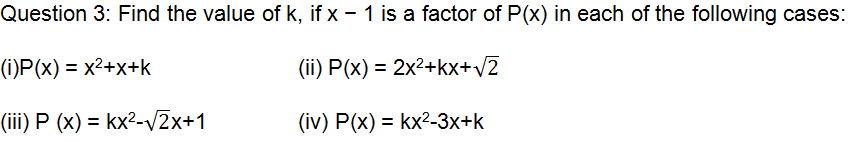Solution:

: If x − 1 is a factor of polynomial p(x), then P(1) must be 0.

(i) P(x) = x2+ x + k

P(1) = (1)2+ 1 + k

= 1+1+

= 2+k

k  =−2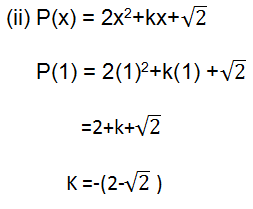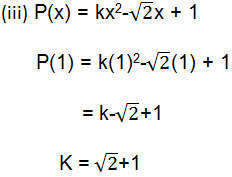(iv) P(x) = kx2-3x + k

P(1) = k(1)2-3(1) + k

= k-3+k

2k = 3

K = 3/2

Question 4: Factorise:

(i) 12x2− 7x + 1                           (ii) 2x2+ 7x + 3

(iii) 6x2+ 5x – 6                           (iv) 3x2− x − 4

Solution:

(i) 12x2− 7x + 1 we can find two numbers,

Such that pq = 12 × 1 = 12 and p + q = −7.

They are p = −4 and q = −3

Here, 12x2− 7x + 1

= 12x2− 4x − 3x + 1

= 4x (3x − 1) − 1 (3x − 1)

= (3x − 1) (4x − 1)

(ii) 2x2+ 7x + 3 we can find two numbers such that pq = 2 × 3= 6 and p + q = 7.

They are p = 6 and q = 1.

Here, 2x2 + 7x + 3

= 2x2+ 6x + x + 3

= 2x (x + 3) + 1 (x + 3)

= (x + 3) (2x+ 1)

(iii) 6x2+ 5x − 6 we can find two numbers such that pq = −36 and p + q = 5.

They are p = 9 and q = −4.

Here, 6x2+ 5x – 6

= 6x2+ 9x − 4x – 6

= 3x (2x + 3) − 2 (2x + 3)

= (2x + 3) (3x − 2)

(iv)  3x2− x − 4 we can find two numbers,

such that pq = 3 × (−4) = −12 and p + q = −1.

They are p = −4 and q = 3

Here, 3x2− x − 4

= 3x2− 4x + 3x – 4

= x (3x − 4) + 1 (3x − 4)

= (3x − 4) (x + 1)

Question 5. Factorize:

(i) x3− 2x2− x + 2                 (ii) x3+ 3x2−9x − 5

(iii) x3+ 13x2+ 32x + 20         (iv) 2y3+ y2− 2y – 1

Solution:

(i) Let P(x) = x3− 2x2− x + 2 all the factor are there. These are ± 1, ± 2.

By trial method, P (1) = (1)3− 2(1)2− 1 + 2

= 1 − 2 − 1+ 2

= 0 Therefore, (x − 1) is factor of polynomial p(x)

Let us find the quotient on dividing x3− 2x2− x + 2 by x − 1.

By long division method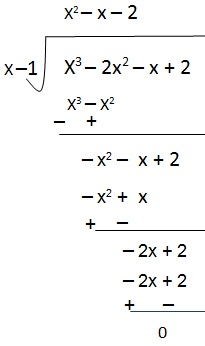Now,

Dividend = Divisor × Quotient + remainder

x3− 2x2− x + 2 = (x – 1) ( X2– x – 2) + 0

= (x – 1) (x2–2x+x–2)

= (x – 1) [x (x–2) + 1(x–2)]

= (x – 1) (x + 1) (x – 2)

(ii)   Let p(x) = x3 – 3x2−9x – 5 all the factor are there. These are ± 1, ± 2.

By trial method, p (–1) = (–1)3– 3(1)2− 9(1) – 5

= –1– 3–9–5     =0

Therefore (x+1) is the factor of polynomial p(x).

.   Let us find the quotient on dividing x3– 3x2−9x – 5 by x+1.

By long division method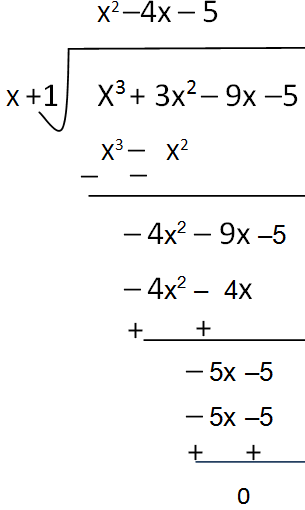Now,

Dividend = Divisor × Quotient + remainder

x3– 3x2−9x – 5 = (x +1) ( X2–4x – 5) + 0

=(x + 1) (x2–5x+x–5)

=(x + 1) [x (x–5) +1(x–5)]

=(x + 1) (x + 1) (x – 5)

(iii)  Let p(x) = x3+ 13x2+ 32x + 20    all the factor are there.

These are ± 1, ± 2, ± 3, ± 4.

By trial method, p (–1) = (–1)3+13(–1)2+ 32(–1) +20

= –1+13–32+20   = 0

Therefore (x+1) is the factor of polynomial p(x).

Let us find the quotient on dividing x3+ 13x2+ 32x + 20 by x+1

By long division method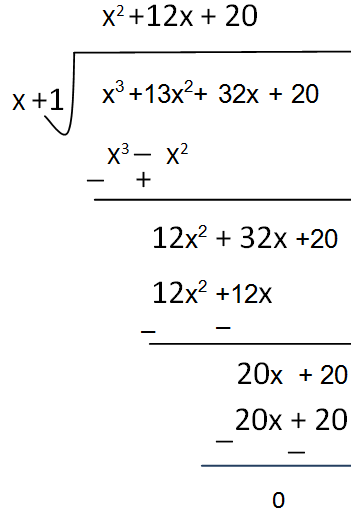Now,

Dividend = Divisor × Quotient + remainder

x3 +13x+ 32x + 20 = (x +1) ( x2 + 12x + 20) + 0

=(x + 1) (x2+10x+2x+20)

=(x + 1) [x (x+10) +2(x+10)]

=(x + 1) (x + 2) (x + 10)

(iv) Let p(y) = 2y3+ y2− 2y – 1 all the factor are there. These are ± 1, ± 2.

By trial method, p (1) =2(1)3 + (1)2 – 2(1) – 1

=2 + 1 – 2 – 1    =0

Therefore (y–1) is the factor of polynomial p(y).

Let us find the quotient on dividing 2y3+ y2− 2y – 1 by y–1.

By long division methodNow,

Dividend = Divisor × Quotient + remainder

2y3+ y2− 2y −1 =(y − 1) (2y2+3y + 1)

= (y − 1) (2y2+2y

= (y − 1) [2y (y+1) + 1 (y + 1)]

= (y − 1) (y + 1) (2y + 1)

##### Other Pages of this Chapter: 2. Polynomials

Important Study materials for classes 06, 07, 08,09,10, 11 and 12. Like CBSE Notes, Notes for Science, Notes for maths, Notes for Social Science, Notes for Accountancy, Notes for Economics, Notes for political Science, Noes for History, Notes For Bussiness Study, Physical Educations, Sample Papers, Test Papers, Mock Test Papers, Support Materials and Books.Mathematics Class - 11th

NCERT Maths book for CBSE Students.

books

## Study Materials List:

##### Solutions ⇒ Class 9th ⇒ Mathematics
1. Number Systems
2. Polynomials
3. Coordinate Geometry
4. Linear Equation In Two Variables
5. Introduction To Euclid’s Geometry
6. Lines and Angles
7. Triangles